Journal homepage ISSN: 1303 - 2968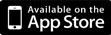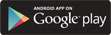©Journal of Sports Science and Medicine (2010) 09, 393 - 397 Research article The Efficiency of Tennis Doubles Scoring Systems Geoff Pollard1, Graham Pollard2,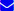Author Information 1 Faculty of Life and Social Sciences, Swinburne University of Technology, Melbourne, Australia 2 Faculty of Information Sciences and Engineering, University of Canberra, Australia Graham Pollard✉ Faculty of Information Sciences and Engineering, University of Canberra, ACT 2601, AustraliaEmail: graham@foulsham.com.au Publish Date Received: 02-02-2010 Accepted: 18-05-2010 Published (online): 01-09-2010 × Geoff Pollard, Graham Pollard. (2010) The Efficiency of Tennis Doubles Scoring Systems. Journal of Sports Science and Medicine (09), 393 - 397.  Email link to this article × Geoff Pollard, Graham Pollard. (2010) The Efficiency of Tennis Doubles Scoring Systems. Journal of Sports Science and Medicine (09), 393 - 397. Your name: Your E-mail: Recipient’s E-mail:
 Abstract Introduction Methods Results Discussion Author biography References

ABSTRACT

In this paper a family of scoring systems for tennis doubles for testing the hypothesis that pair A is better than pair B versus the alternative hypothesis that pair B is better than A, is established. This family or benchmark of scoring systems can be used as a benchmark against which the efficiency of any doubles scoring system can be assessed. Thus, the formula for the efficiency of any doubles scoring system is derived. As in tennis singles, one scoring system based on the play-the-loser structure is shown to be more efficient than the benchmark systems. An expression for the relative efficiency of two doubles scoring systems is derived. Thus, the relative efficiency of the various scoring systems presently used in doubles can be assessed. The methods of this paper can be extended to a match between two teams of 2, 4, 8, …doubles pairs, so that it is possible to establish a measure for the relative efficiency of the various systems used for tennis contests between teams of players.

Key words: Efficiency of tennis scoring systems, play-the-loser, play-the-winner, volleyball, team tennis

Key Points
• A relatively straightforward expression or formula for the efficiency of a tennis doubles scoring system has been established.
• The expression for the relative efficiency of two tennis doubles scoring systems is a simple one, and is the same as that for two singles scoring systems.
• The methodology of this paper can be used and extended so that the efficiency of a scoring system for a match between two teams of doubles pairs can be measured.

 INTRODUCTION

In a very elegant paper, Miles, 1984 noted the link between sports scoring systems and sequential statistical hypothesis testing, and the following few paragraphs outline the essential features of his contribution to scoring systems in that paper. One important characteristic of a scoring system is its efficiency. (Other important characteristics include the mean, the variance, and the skewness of the number of points played, and the probability that the better player or better team wins when that scoring system is used.) If two scoring systems SS1 and SS2 have the same probability of correctly identifying the better player, SS1 is said to be more efficient than SS2 if it has a smaller expected number of points played. Here we identify how the efficiency (a simple numerical value) of a scoring system can be determined.

Unipoints

Many sports consist of playing a sequence of points each of which is won by either player A or player B. If there is only one type of point in the match, we have unipoints in which p (q) is the probability player A wins (loses) each point (p + q = 1). Player A is the better player if p > 0.5. Miles, 1984 considered fair scoring systems possessing sensible regularity conditions and only one type of point, which he called uniformats.

For testing the hypothesis H0: Player A better than player B, versus the alternative hypothesis H1: Player B better than player A,

he applied the result of Wald and Wolfowitz (1948) to conclude that there is a unique class of optimally efficient uniformats, given by the Sequential Probability Ratio Test, and that it is {Wn} (n = 1,2,3,…) where Wn is the uniformat in which the winner is the first player to achieve a lead of n points over his opponent. The key characteristics of Wn are given by

and the efficiency of a general uniformat with key characteristics P and μ is given by

Bipoints

If there are two types of points (a-points when player A serves, and b-points when player B serves), we have bipoints. The probability player A wins a point on service is pa, and the probability player B wins a point on service is pb. Player A is the better player if pa > pb. For the bipoints situation, Miles, 1984 considered fair scoring systems with sensible regularity conditions, which he called biformats.

Noting that Wald (1947) had recommended the use of paired trials for comparing two binomial probabilities (H0: pa > pb Vs H1: pb > pa), Miles (1984) set up {Wn(point-pairs)}, where n = 1, 2, 3, …, as the standard biformat family of scoring systems (with unit efficiency) against which the efficiency of any tennis singles scoring system could be measured. An example of this is given by Pollard and Noble (2004). Here, point-pairs represent the playing of pairs of points consisting of an a-point and a b-point.

Miles (1984) showed that the efficiency of a bipoints scoring system with mean μ and probability P that the better player wins, is given by

Using the play-the-loser mechanism (PL) in which a win by player A (B) is followed by a b- (a-) point, Miles (1984) showed that the family of reversal biformats {W1(WnPLa,WnPLb)} (n = 2, 3, 4, …) was slightly more efficient than {Wn(point-pairs)} (n = 1, 2, 3, …) when pa + pb > 1, which is the tennis context. Pollard (1992) showed that this PL family of scoring systems was not only more efficient than the AL or point-pairs family of systems, but that it (and its stochastic equivalent, for example, one based on PL generalized point-pairs) was the most efficient possible. (Note that when using PL generalized point-pairs, a win to A (AA) is followed by a (b, b) point-pair, a draw (AB) is followed by an (a, b) point-pair, and a loss to A (BB) is followed by an (a, a) point-pair.) He showed correspondingly that the family of play-the-winner (PW) reversal biformats {W1(WnPWa,WnPWb)} (n = 2, 3, 4, …) is optimally efficient when pa + pb < 1 (which is the volleyball situation).

If Pk (Qk) denotes the probability that player A wins (loses) in k points, then a scoring system is said to have the constant probability ratio property (c.p.r.) if Pk/Qk is constant for all k for which Qk > 0. Pollard (1992) showed that the above families of AL, PL and PW scoring systems possessed the c.p.r. property.

In tennis doubles, we have four probabilities, pa1, pa2, pb1 and pb2 where pa1 is player A1’s probability of winning a point on service, pa2 is player A2’s probability of winning a point on service, pb1 is player B1’s probability of winning a point on service, and pb2 is player B2’s probability of winning a point on service. Thus, it is natural to call this situation quadpoints. Using these four probabilities, Pollard, 2005 showed that a first to six games rule for a set of tennis could be unfair in some doubles situations, as could the present ‘first to seven’ and ‘first to ten’ tiebreak games rules. Also, Pollard (1990) carried out research on the asymptotic efficiencies of some quadpoints Wn systems (for large n). He noted that some complex Wn systems could be decomposed into smaller independent components called modules, which could in turn be analyzed to produce approximate asymptotic values for P, μ and ρ for the whole system. In a natural extension of point-pairs, he set up a basic module consisting of the 4 points {a1, b1, a2, b2}, which we call ‘point-quads’. He also considered a second module, called PLteams, using PL and generalized point-pairs in the following way. The first section of the PLteams module starts with an (a1, b1) point-pair, and the PL mechanism operates in the following way. A point-pair win to pair A, (AA), is followed by a (b1, b2) point-pair whilst a point-pair win to pair B, (BB), is followed by an (a1, a2) point-pair. The first section of the module finishes as soon as a draw, (AB), occurs, and the second section of the module begins with a point-pair (a2, b2), and proceeds in the same manner as above until a draw occurs, thus completing the whole module. He showed that, provided the modulus of (pa1 - pa2) equals the modulus of (pb1 - pb2), PLteams (PWteams) modules used in conjunction with Wn systems (n large) are asymptotically more efficient than the {Wn(point-quads)} (n large) system when ((pa1+pa2)/2 + (pb1+pb2)/2) is greater (less) than 1. Note that the situation when ((pa1+pa2)/2 + (pb1+pb2)/2) is greater than 1 is referred to as the ‘tennis context’ since it corresponds to the typical advantage of serving (rather than receiving) in tennis.

In this paper some quadpoints results are established for non-asymptotic cases.

 METHODS

It was noted above that in doubles we have four service probabilities pa1, pa2, pb1 and pb2. In order to establish the efficiency of a doubles scoring system we need to set up an appropriate structure so that P and μ do not depend on the order of the four points being played. This suggests setting up the family of scoring systems {Wn(W1(W2AL(a1, b1), W2AL(a2, b2)), W1(W2AL(a1, b2), W2AL(a2, b1)))} (n = 1, 2, 3, …) as the standard scoring system against which the efficiency of any doubles scoring system can be measured. Here, for example, AL(a1, b1) represents the playing of alternating a1 and b1 points. Note that AL(a1, b1) is stochastically equivalent to (a1, b1) point-pairs when used with Wn (n even).

An expression for the efficiency of a doubles scoring system is now derived. Firstly we note that the above scoring system has four components, each with an AL structure. The component listed first, W2AL(a1, b1) has characteristics P1, μ1 and Q1 given by

It is noted that W2AL(a1, b1) has the c.p.r. property, and that μ1 is equal to the mean number of points conditional on pair A winning, and is also equal to the mean number of points conditional on pair A losing (Pollard, 1992). This fact is used in the following analysis.

Corresponding expressions for P2, Q2 and μ2 for the second component can be written down.

The first half of the above scoring system, W1(W2AL(a1, b1), W2AL(a2, b2)), is now analysed. This first half of the scoring system amounts to playing W2AL(a1, b1) and W2AL(a2, b2) until one pair wins both components. If these two components are won by different pairs, the process is repeated until one pair wins both. It can be shown that W1(W2AL(a1, b1), W2AL(a2, b2)) has the c.p.r. property. The probability pair A wins this half of the above scoring system is given by

and the expected duration of this first half is given by

where R1,2 is the probability that the pairs each win one component of this half, and is given by

The second half of the above scoring system can be analyzed similarly giving corresponding results. The subscipts 3 and 4 are used for this second half. Combining the results for the two halves of the scoring system, we have

Thus, for the complete scoring system (general n), which also possesses the c.p.r. property, the probability pair A wins, Pn, and the mean number of points played, μn , satisfy the equations

The efficiency of a doubles scoring system with mean μ and probability pair A wins equal to P, is now considered. The efficiency is equal to μn/μ where μn is the mean of the above standard scoring system with the same P value (i.e. P = Pn). Noting

and the above expression for μn , the efficiency ρ of a doubles scoring system with probability P and mean μ is given by

Expressing μ1,2,3,4 and (P1,2,3,4Q1,2,3,4) as functions of pa1, pa2, pb1 and pb2, we have, after some algebra,

This is the general expression for the efficiency of a doubles scoring system. Note that when pa1 = pa2 = pa and pb1 = pb2 = pb, this expression is equal to the expression given in the introduction for the efficiency of tennis singles scoring systems.

Thus, it can be seen that when comparing two doubles scoring systems, we simply need to compare their respective values for the expression ((P – Q)*ln(P/Q))/μ, known as the relative efficiency, as the rest of the expression for ρ above is simply a function of the parameters for the players. Thus, interestingly, this result for unipoints and bipoints carries over to the quadpoints situation.

The efficiency of {W(a1, a2, b1, b2)}

The efficiency of the Wn systems using sets of the four points at a time, called point-quads, is considered. (Note that this system involves playing sets of 4 points, and then making a decision as to whether pair A has won or lost, or the match continues). This system is a natural extension of point-pairs to the quadpoints case, and we might expect it to have unit efficiency, as in the point-pairs case. As in the paper by Pollard, 1990, this set of four points is called a module. The independent modules effectively become the steps of a general one-dimensional walk in discrete time. Using the approach and notation of Cox and Miller, 1965, the steps in the random walk, Zi , are mutually independent random variables on the integers …, -2, -1, 0, 1, 2,… and the moment generating function (m.g.f.) of Zi is defined by

If P(Q) represent the probability of absorption in states [a, infinity) ((-infinity, -b]), and E(N) is the expected number of steps to absorption, then, neglecting the excess over the barriers,

Using the above expressions for the ratio P/Q and E(N), and representing Wn(point-quads) as system 1, and the above standard system as system 2, we have

It turns out that the efficiency of Wn(point-quads) (n = 1, 2, 3, …) is slightly less than unity, even when n is large. For example, when pa1 = 0.9, pa2 = 0.8, pb1 = 0.7, and pb2 = 0.6, it can be shown that E(D1) = 4, E(S1) = 0.8, and exp(θ1) = 1.7650, and E(D2) = 39.7541, E(S2) = 7.8502, and exp(θ2) = 1.7908 (note that exp(4θ2) = (pa1pa2qb1qb2)/(pb1pb2qa1qa2)), and it follows that, when n = 30 say, ρ1/ ρ2 = 0.9876, which is slightly less than 1. A few differences between systems 1 and 2 (or their modules) are noted here. A module of system 2 has the same expected number of a- and b- points in total, but the expected number of a1 points is not equal to the expected number of a2 points, and the expected number of b1 points is not equal to the expected number of b2 points (For example, these are 10.3159, 9.5611, 10.8192 and 9.0579 respectively in the above example). Also system 1 can have excesses of 1, 2 or 3 over the boundaries, whereas system 2 cannot have any excesses.

The efficiency of some PL and PW quadpoints systems

In this section we consider whether the PL and PW service exchange mechanisms can be used to find super-efficient scoring systems for quadpoints, as has been noted was possible in the case of bipoints. We firstly consider {Wn(W1(W2mPL(a1, b1), W2mPL(a2, b2)), W1(W2mPL(a1, b2), W2mPL(a2, b1)))} (n = 1, 2, 3, …; m = 1, 2, 3, …). Note that W2mPL(a1, b1), for example, is the generalized PL point-pair structure described earlier that makes use of the W2m stopping rule.

For the first of the four components, W2mPL(a1, b1), we have, using a similar notation to above,

Similar expressions for the other three components can be written down. Firstly, the case when n = 1 is considered. Using similar methods to above, expressions for P1,2,3,4 and μ1,2,3,4 can be determined for this PL scoring system, and it can be shown by substituting these expressions into the formula for ρ above that the efficiency of this PL system is less than unity for all m = 2, 3, 4, …. For example, Table 1 has values for the efficiency of this PL system when n = 1 and m = 1, 2, 3, 4 and 5, when pa1 = 0.9, pa2 = 0.8, pb1 = 0.7 and pb2 = 0.6. This table also gives values for the efficiency of the corresponding alternating and play-the-winner scoring systems {Wn(W1(W2mAL(a1, b1), W2mAL(a2, b2)), W1(W2mAL(a1, b2), W2mAL(a2, b1)))} (n = 1, 2, 3, …; m = 1, 2, 3, …) and {Wn(W1(W2mPW(a1, b1), W2mPW(a2, b2)), W1(W2mPW(a1, b2), W2mPW(a2, b1)))} (n = 1, 2, 3, …; m = 1, 2, 3, …), for the cases when n = 1 and m = 1, 2, 3, 4 and 5.

It was observed that the efficiency of all of these systems (when n = 1) is less than unity as soon as m is greater than unity. Noting that these scoring systems with general n amount to playing the corresponding system with n = 1 n or more times, it is clear that the efficiency of these systems when n is greater than 1 (and m is not unity) must also be less than unity. This follows since efficiency under nesting is essentially multiplicative (Miles, 1984). Thus, it follows that it is not possible to use these PL and PW mechanisms in the above manner to increase efficiency above 1.

The efficiency of some other PL and PW quadpoints systems

We now consider an alternative application of the PL mechanism, and consider the PLteams module approach mentioned in the introduction. For example, for this module as defined, when pa1 = 0.9, pa2 = 0.8, pb1 = 0.7 and pb2 = 0.6, it was shown numerically that θ was approximately 0.8642 and E(D)/E(S) was 7.5 for each ‘half’ of the module. (It was verified numerically that E(D)/E(S) for this module is in general equal to (pa1 + pa2 + pb1 + pb2)/(pa1 + pa2 – pb1 – pb2). The value for θ, however, needed to be calculated numerically.) Thus, for these parameter values, E(D2)/(θ2E(S2)) is equal to 8.6909 (from above), and E(D1)/(θ1E(S1)) is equal to 8.6785, so the ratio is 1.0014. When n = 30, exp(exp(-nθ2)-exp(-nθ1)) = 1.0000, so the efficiency of this W30(PLteams) relative to the standard system is equal to 1.0014 when pa1 = 0.9, pa2 = 0.8, pb1 = 0.7 and pb2 = 0.6. Thus, in the tennis context, for quadpoints just as for bipoints, it is possible to use the PL mechanism to achieve efficiencies greater than 1. It is noted that the PLteams module as defined in the introduction should be extended so that it includes two additional components beginning with (a1, b2) and (a2, b1). Pollard, 1990 noted that the values of θ0 and of E(D)/E(S) were unaffected by reversing the roles of b1 and b2; namely the case in which the first section of the module started with an (a1, b2) point-pair and the second section with an (a2, b1) point-pair. Thus, as in the bipoints case, any increases in efficiency above 1 are miniscule. Correspondingly, when the underlying p-values are less than 0.5 as in volleyball, the PW mechanism can be used to achieve efficiencies marginally greater than 1.

The above module, PLteams, made use of point-pairs. However, a corresponding module making use of points rather than point-pairs can be formed. This is done by using the PL mechanism between teams but alternating the points within each team. It was verified that the value of E(D)/E(S) was again given by (pa1 + pa2 + pb1 + pb2)/(pa1 + pa2 – pb1 – pb2), and that exp(θ0) was the square root (since points rather than point-pairs are involved) of its value for the PLteams module. Thus, these two modules are equivalent. The advantage of this formulation, however, is that it can be extended to multi-points (a1, a2, a3, …; b1, b2, b3, …) by ‘rotating’ or ‘cycling’ through the relevant points rather than alternating between the pair of them. This result is relevant to the situation where there are two teams of two doubles pairs (i.e. a1, a2, a3, a4; b1, b2, b3, b4), even though ‘rotating’ or ‘cycling’ would be impractical as it would involve (amongst other things) players going on and off the court continuously. Nevertheless, a relative measure of efficiency in this team situation could be evaluated. It can be seen that this paragraph is related to the next section.

An extension of the quadpoints systems

Suppose team A has two doubles pairs represented by [(a1, a2), (a3, a4)] and team B has two doubles pairs represented by [(b1, b2), (b3, b4)]. Then, denoting the above quadpoints standard scoring system {W1(W1(W2AL(a1, b1), W2AL(a2, b2)), W1(W2AL(a1, b2), W2AL(a2, b1)))} by SS(a1, a2; b1,b2), it follows that Wn(W1(SS(a1, a2; b1,b2), SS(a3, a4; b3,b4)), W1(SS(a1, a2; b3,b4), SS(a3, a4; b1,b2))) (n = 1, 2, 3,…) has the c.p.r. property, and is the corresponding standard family of scoring systems for two teams with two doubles pairs per team. Thus, it can be used as the standard family of scoring systems against which to assess the efficiency of team doubles with two pairs per team. In the same way, this process can be extended to teams of 4 doubles pairs, 8 doubles pairs, etcetera.

 RESULTS

A family of doubles scoring systems has been identified and used to establish a framework for measuring the efficiency of any tennis doubles scoring system. An expression for the efficiency of a general tennis doubles scoring system with one parameter for each player has been derived. Further, the relative efficiency expression in this doubles context is in fact the same as that for tennis singles, and the same as for squash.

A doubles scoring system based on simply playing groups of the four point-types at a time and using the Wn stopping rule has been shown to have slightly smaller efficiency than the framework scoring systems.

Two scoring systems using the PL rule have been considered. In the tennis context, one has efficiency less than the framework family of scoring systems, and the other has efficiency greater than that family.

It has been demonstrated how the methods of this paper can be further developed to find the efficiency of scoring systems used in a contest between two teams of 2, 4, 8, …doubles pairs.

 CONCLUSION

In tennis doubles pair A has probabilities pa1 and pa2 of winning a point on service when players A1 and A2 serve respectively, and correspondingly pair B has probabilities pb1 and pb2. In this paper a family of standard scoring systems for testing the hypothesis that pair A is better than B versus the alternative hypothesis that pair B is better than A, for this four parameter situation, has been established. Characteristics of this family of scoring systems such as the probability that each pair wins and the expected number of points played do not depend on the order of the four types of points played. A formula for the efficiency of any doubles scoring system relative to this family of scoring systems has been determined. Thus, this scoring system can be used as a benchmark against which the efficiency of any doubles scoring system can be evaluated. This is particularly useful as there is a range of scoring systems presently used for doubles. The standard family of scoring systems that has been set up is very efficient. It has been shown that, as in singles tennis with two parameters rather than four, there is a family of ever-so-slightly more efficient systems that make use of the play-the-loser service exchange mechanism. As in singles, this super-efficient family of play-the-loser scoring systems is of theoretical rather than sporting relevance.

An expression for the relative efficiency of two doubles scoring systems has been identified, and it has been shown to be identical to that for tennis singles and other sports such as squash which typically need just one parameter when modelling.

The methods of this paper can be applied to a match between two teams of 2, 4, 8, etc. doubles pairs. That is, it is possible to establish a yardstick, and to measure the efficiency of such team contests.

AUTHOR BIOGRAPHYGeoff Pollard Employment: President, Tennis Australia, Melbourne, Australia and PhD student, Swinburne University, Melbourne, Australia. Degree: MSc in mathematical statistics. Research interests: Mathematical statistics applied to sport, demographic studies. E-mail: gpollard@tennis.com.auGraham Pollard Employment: Emeritus Professor (Statistics), University of Canberra, Australia. Degree: PhD. Research interests: Scoring systems in sport and in assessment. Optimal learning. E-mail: graham@foulsham.com.au

REFERENCES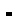Cox D., Miller H (1965) The theory of stochastic processes. London. Chapman and Hall.Miles R (1984) Symmetric sequential analysis: the efficiencies of sports scoring systems (with particular reference to those of tennis). Journal of the Royal Statistical Society B 46, 93-108.Pollard G (1990) A method for determining the asymptotic efficiency of some sequential probability ratio tests. Australian Journal of Statistics 32, 191-204.Pollard G (1992) The optimal test for selecting the greater of two binomial probabilities. Australian Journal of Statistics 34, 273-284.Pollard G (2005) On solving an aspect of unfairness in the tennis doubles scoring system. Chance 18, 17-19.Pollard G., Noble K., Morton R., Ganesalingam S (2004) Proceedings of the Seventh Australasian Conference on Mathematics and Computers in Sport. The benefits of a new game scoring system in tennis: the 50-40 game. New Zealand. Massey University.Wald A (1947) Sequential analysis. New York. Wiley.Wald A., Wolfowitz J (1948) Optimum character of the sequential probability ratio test. Annals of Mathematical Statistics 19, 326-339.

 Home Issues About Authors Contact Current Editorial board Authors instructions Email alerts In Press Mission For Reviewers Archive Scope Supplements Statistics Most Read Articles Most Cited Articles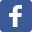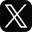This work is licensed under a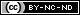Creative Commons Attribution-NonCommercial-NoDerivatives 4.0 International License.